# Projection of Lines

Mar. 14, 2017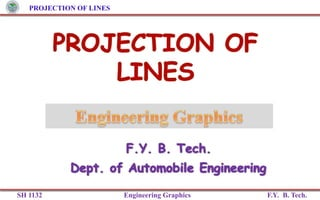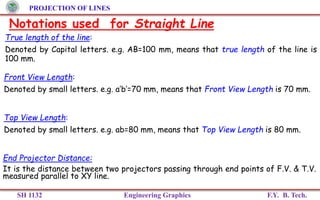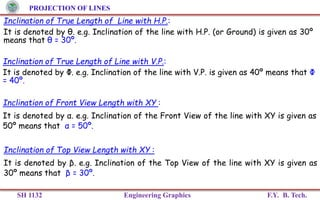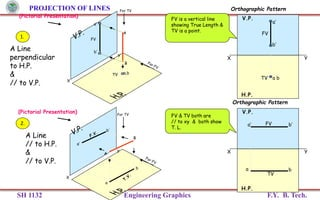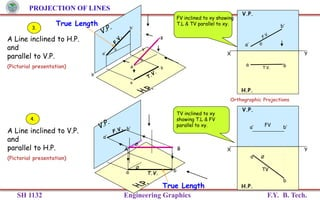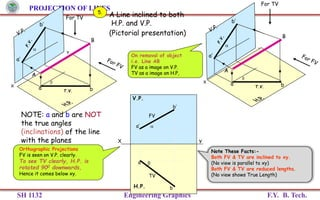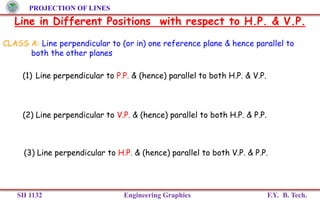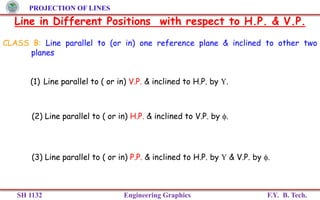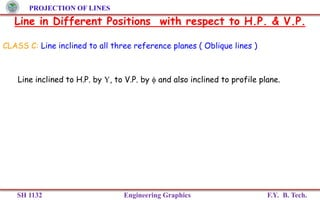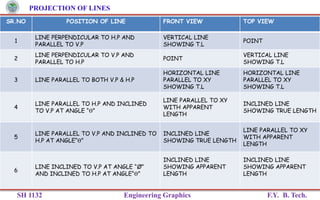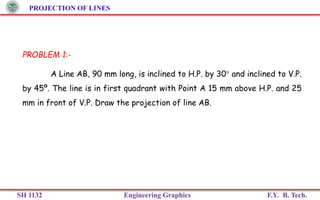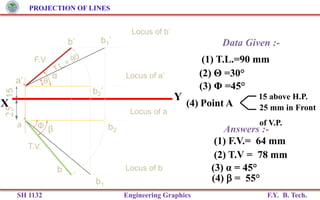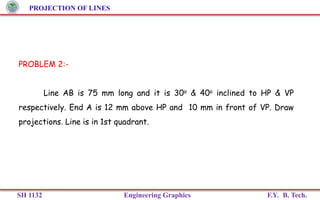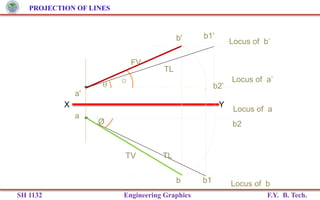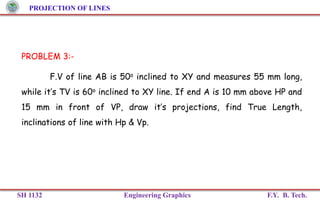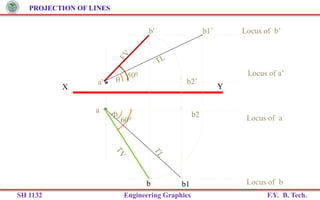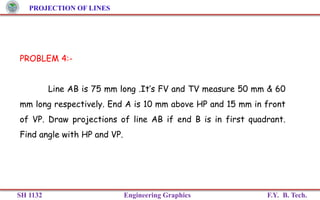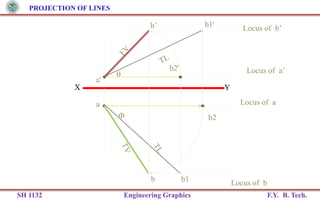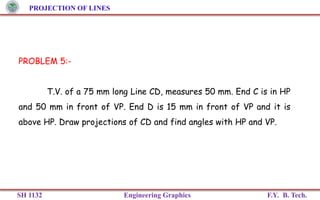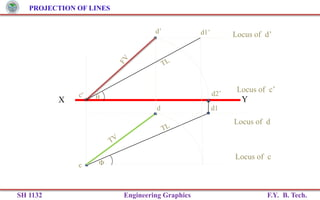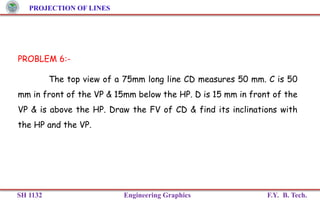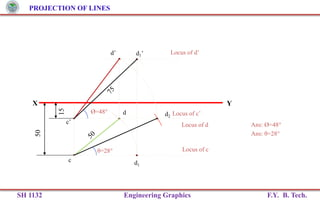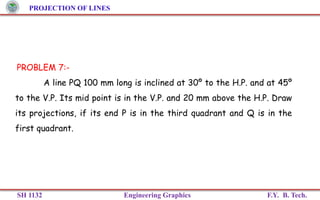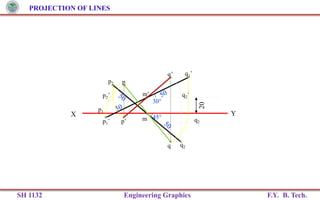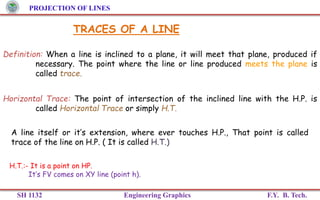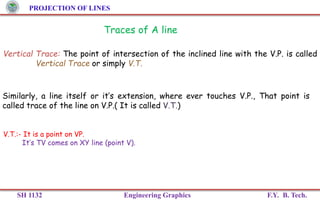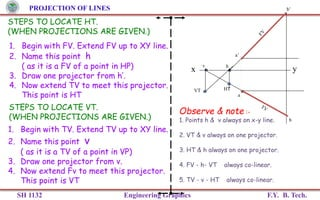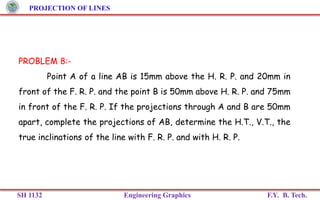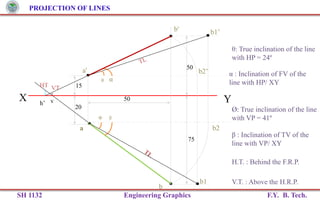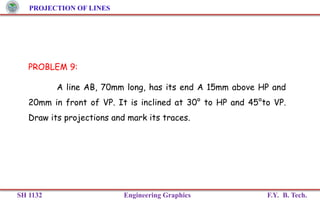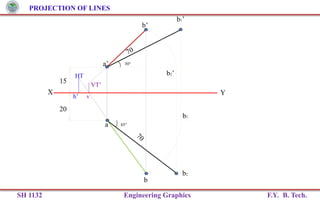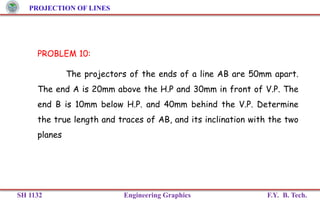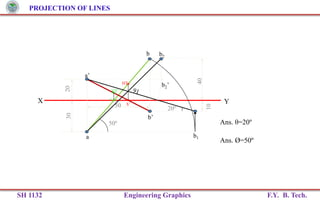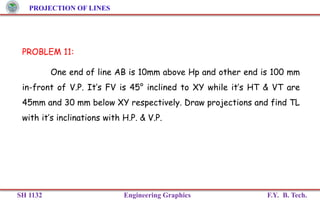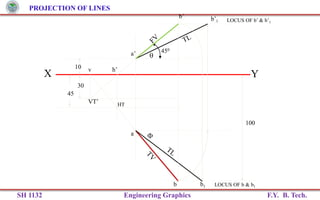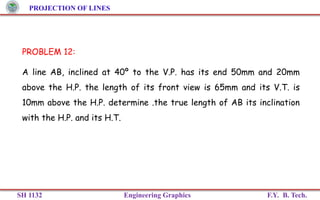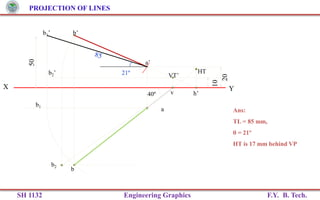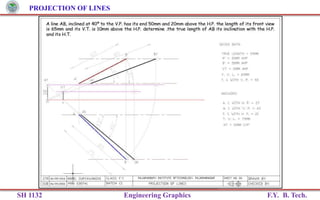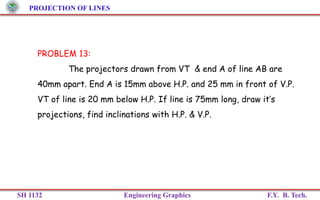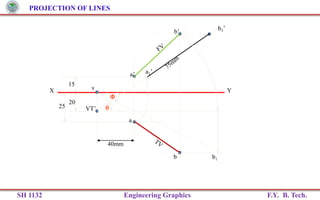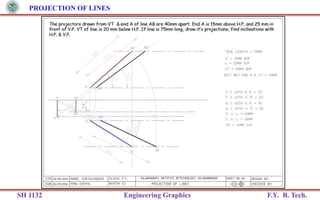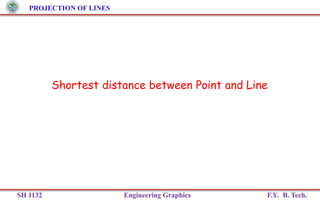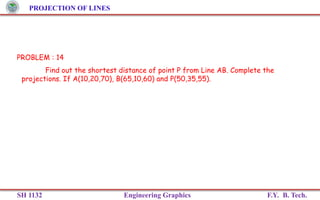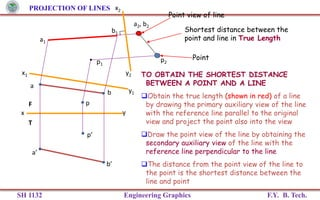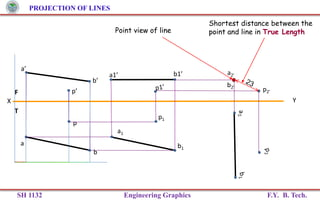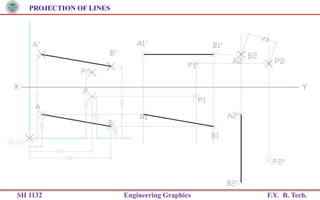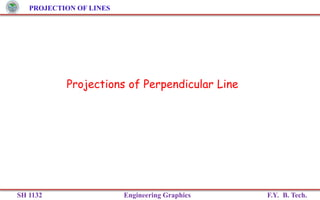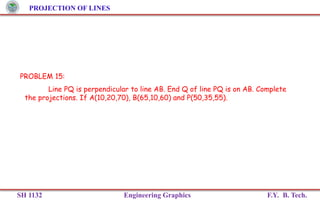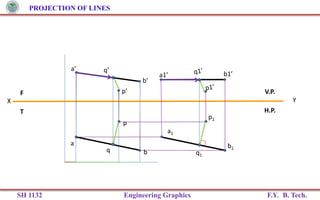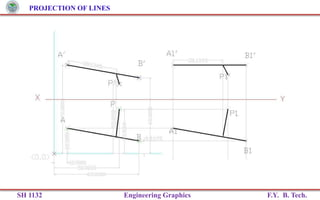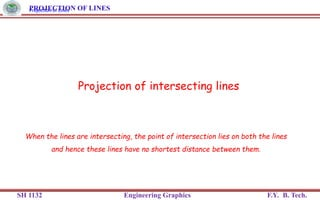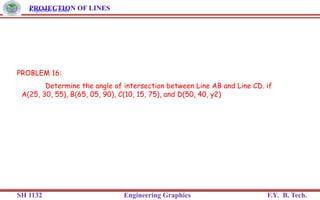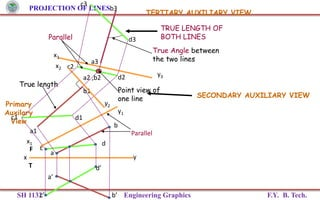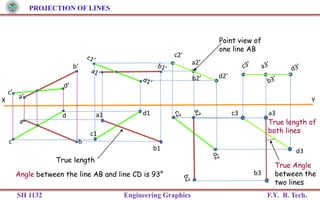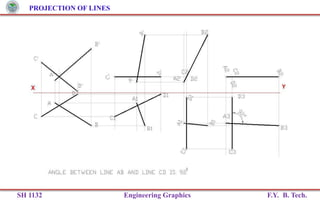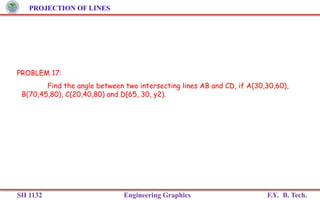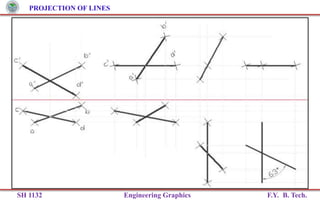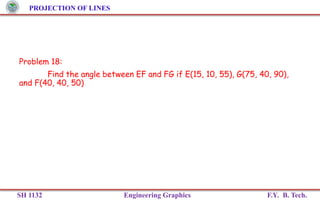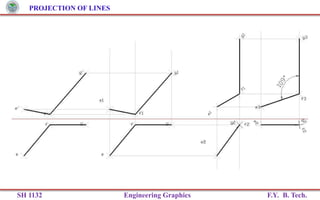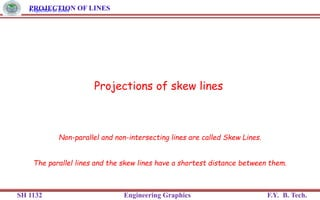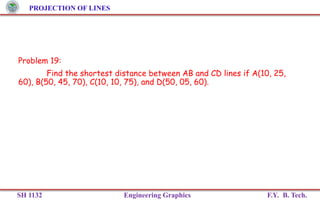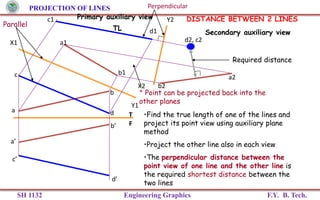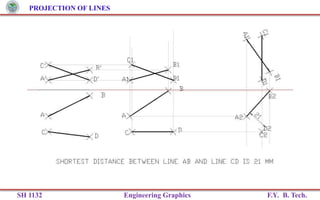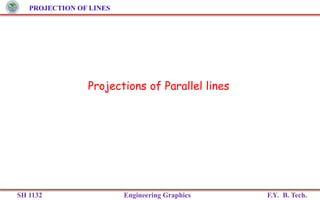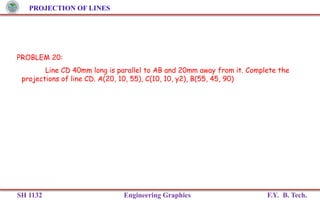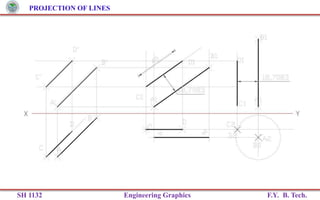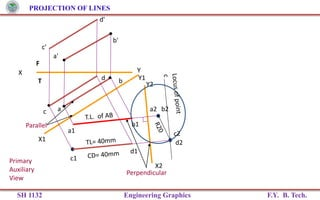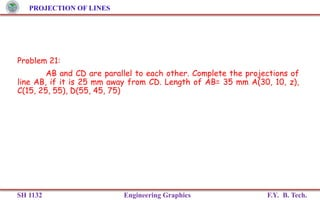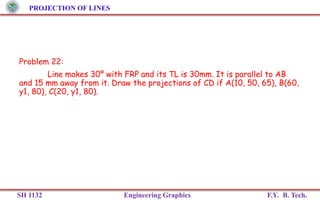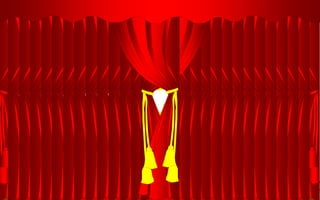1 of 71

### Projection of Lines

• 1. PROJECTION OF LINES SH 1132 Engineering Graphics F.Y. B. Tech.
• 2. PROJECTION OF LINES SH 1132 Engineering Graphics F.Y. B. Tech. Notations used for Straight Line True length of the line: Denoted by Capital letters. e.g. AB=100 mm, means that true length of the line is 100 mm. Front View Length: Denoted by small letters. e.g. a’b’=70 mm, means that Front View Length is 70 mm. Top View Length: Denoted by small letters. e.g. ab=80 mm, means that Top View Length is 80 mm. End Projector Distance: It is the distance between two projectors passing through end points of F.V. & T.V. measured parallel to XY line.
• 3. PROJECTION OF LINES SH 1132 Engineering Graphics F.Y. B. Tech. Inclination of Front View Length with XY : It is denoted by α. e.g. Inclination of the Front View of the line with XY is given as 50º means that α = 50º. Inclination of True Length of Line with V.P.: It is denoted by Φ. e.g. Inclination of the line with V.P. is given as 40º means that Φ = 40º. Inclination of True Length of Line with H.P.: It is denoted by θ. e.g. Inclination of the line with H.P. (or Ground) is given as 30º means that θ = 30º. Inclination of Top View Length with XY : It is denoted by β. e.g. Inclination of the Top View of the line with XY is given as 30º means that β = 30º.
• 4. PROJECTION OF LINES SH 1132 Engineering Graphics F.Y. B. Tech. X Y X Y b’ a’ b a a,b a’ b’ B A TV FV A B X Y H.P. V.P. a’ b’ a b FV TV X Y H.P. V.P. a b a’ b’FV TV For TV For TV FV is a vertical line showing True Length & TV is a point. FV & TV both are // to xy & both show T. L. 1. 2. A Line perpendicular to H.P. & // to V.P. A Line // to H.P. & // to V.P. Orthographic Pattern Orthographic Pattern (Pictorial Presentation) (Pictorial Presentation)
• 5. PROJECTION OF LINES SH 1132 Engineering Graphics F.Y. B. Tech. A Line inclined to H.P. and parallel to V.P. (Pictorial presentation) X Y A B b’ a’ b a   A Line inclined to V.P. and parallel to H.P. (Pictorial presentation) Ø a b a’ b’ BA Ø X Y H.P. V.P. T.V. a b a’ b’  X Y H.P. V.P. Øa b a’ b’ TV FV TV inclined to xy showing T.L & FV parallel to xy. 3. 4. FV inclined to xy showing T.L & TV parallel to xy. Orthographic Projections True Length True Length
• 6. PROJECTION OF LINES SH 1132 Engineering Graphics F.Y. B. Tech. X Y a’ b’ a b B A   For TV T.V. X Y a’ b’ a b   T.V. For TV B A X Y   H.P. V.P. a b FV TV a’ b’ A Line inclined to both H.P. and V.P. (Pictorial presentation) 5. Note These Facts:- Both FV & TV are inclined to xy. (No view is parallel to xy) Both FV & TV are reduced lengths. (No view shows True Length) Orthographic Projections FV is seen on V.P. clearly. To see TV clearly, H.P. is rotated 900 downwards, Hence it comes below xy. On removal of object i.e. Line AB FV as a image on V.P. TV as a image on H.P, NOTE: a and b are NOT the true angles (inclinations) of the line with the planes
• 7. PROJECTION OF LINES SH 1132 Engineering Graphics F.Y. B. Tech. Line in Different Positions with respect to H.P. & V.P. CLASS A: Line perpendicular to (or in) one reference plane & hence parallel to both the other planes (1) Line perpendicular to P.P. & (hence) parallel to both H.P. & V.P. (2) Line perpendicular to V.P. & (hence) parallel to both H.P. & P.P. (3) Line perpendicular to H.P. & (hence) parallel to both V.P. & P.P.
• 8. PROJECTION OF LINES SH 1132 Engineering Graphics F.Y. B. Tech. CLASS B: Line parallel to (or in) one reference plane & inclined to other two planes (1) Line parallel to ( or in) V.P. & inclined to H.P. by . (2) Line parallel to ( or in) H.P. & inclined to V.P. by . (3) Line parallel to ( or in) P.P. & inclined to H.P. by  & V.P. by . Line in Different Positions with respect to H.P. & V.P.
• 9. PROJECTION OF LINES SH 1132 Engineering Graphics F.Y. B. Tech. CLASS C: Line inclined to all three reference planes ( Oblique lines ) Line inclined to H.P. by , to V.P. by  and also inclined to profile plane. Line in Different Positions with respect to H.P. & V.P.
• 10. PROJECTION OF LINES SH 1132 Engineering Graphics F.Y. B. Tech. SR.NO POSITION OF LINE FRONT VIEW TOP VIEW 1 LINE PERPENDICULAR TO H.P AND PARALLEL TO V.P VERTICAL LINE SHOWING T.L POINT 2 LINE PERPENDICULAR TO V.P AND PARALLEL TO H.P POINT VERTICAL LINE SHOWING T.L 3 LINE PARALLEL TO BOTH V.P & H.P HORIZONTAL LINE PARALLEL TO XY SHOWING T.L HORIZONTAL LINE PARALLEL TO XY SHOWING T.L 4 LINE PARALLEL TO H.P AND INCLINED TO V.P AT ANGLE “Ѳ” LINE PARALLEL TO XY WITH APPARENT LENGTH INCLINED LINE SHOWING TRUE LENGTH 5 LINE PARALLEL TO V.P AND INCLINED TO H.P AT ANGLE“Ѳ” INCLINED LINE SHOWING TRUE LENGTH LINE PARALLEL TO XY WITH APPARENT LENGTH 6 LINE INCLINED TO V.P AT ANGLE “Ø” AND INCLINED TO H.P AT ANGLE“Ѳ” INCLINED LINE SHOWING APPARENT LENGTH INCLINED LINE SHOWING APPARENT LENGTH
• 11. PROJECTION OF LINES SH 1132 Engineering Graphics F.Y. B. Tech. PROBLEM 1:- A Line AB, 90 mm long, is inclined to H.P. by 30° and inclined to V.P. by 45º. The line is in first quadrant with Point A 15 mm above H.P. and 25 mm in front of V.P. Draw the projection of line AB.
• 12. PROJECTION OF LINES SH 1132 Engineering Graphics F.Y. B. Tech. 2515 Φ α β a’ a b’ b1’ Y X b b2 θ Locus of b’ Locus of b Data Given :- (1) T.L.=90 mm (2) Θ =30° (3) Φ =45° (4) Point A 15 above H.P. 25 mm in Front of V.P. Answers :- (1) F.V.= 64 mm (2) T.V = 78 mm (3) α = 45° (4)  = 55° b2’ b1 . . Locus of a’ Locus of a
• 13. PROJECTION OF LINES SH 1132 Engineering Graphics F.Y. B. Tech. PROBLEM 2:- Line AB is 75 mm long and it is 30o & 40o inclined to HP & VP respectively. End A is 12 mm above HP and 10 mm in front of VP. Draw projections. Line is in 1st quadrant.
• 14. PROJECTION OF LINES SH 1132 Engineering Graphics F.Y. B. Tech. a' b' a b X Y b2’ b1 Ø α TL FV TV b2 Locus of b’ Locus of b  TL b1’ Locus of a’ Locus of a
• 15. PROJECTION OF LINES SH 1132 Engineering Graphics F.Y. B. Tech. PROBLEM 3:- F.V of line AB is 50o inclined to XY and measures 55 mm long, while it’s TV is 60o inclined to XY line. If end A is 10 mm above HP and 15 mm in front of VP, draw it’s projections, find True Length, inclinations of line with Hp & Vp.
• 16. PROJECTION OF LINES SH 1132 Engineering Graphics F.Y. B. Tech. X a' Y a b' 500 b 600 b1 b1’   b2 b2’ Locus of b’ Locus of b Locus of a’ Locus of a
• 17. PROJECTION OF LINES SH 1132 Engineering Graphics F.Y. B. Tech. PROBLEM 4:- Line AB is 75 mm long .It’s FV and TV measure 50 mm & 60 mm long respectively. End A is 10 mm above HP and 15 mm in front of VP. Draw projections of line AB if end B is in first quadrant. Find angle with HP and VP.
• 18. PROJECTION OF LINES SH 1132 Engineering Graphics F.Y. B. Tech. X Y a' a b’ b1 b2' b   b2 b1' Locus of b’ Locus of b Locus of a Locus of a’
• 19. PROJECTION OF LINES SH 1132 Engineering Graphics F.Y. B. Tech. PROBLEM 5:- T.V. of a 75 mm long Line CD, measures 50 mm. End C is in HP and 50 mm in front of VP. End D is 15 mm in front of VP and it is above HP. Draw projections of CD and find angles with HP and VP.
• 20. PROJECTION OF LINES SH 1132 Engineering Graphics F.Y. B. Tech. X Y c' c Locus of d d d1 d’   Locus of d’d1’ d2’ Locus of c Locus of c’
• 21. PROJECTION OF LINES SH 1132 Engineering Graphics F.Y. B. Tech. PROBLEM 6:- The top view of a 75mm long line CD measures 50 mm. C is 50 mm in front of the VP & 15mm below the HP. D is 15 mm in front of the VP & is above the HP. Draw the FV of CD & find its inclinations with the HP and the VP.
• 22. PROJECTION OF LINES SH 1132 Engineering Graphics F.Y. B. Tech. X Y 15 50 c’ c Locus of c’d d1 d1’d’ Ø=48° Ans: Ø=48° d2 θ=28° Ans: θ=28° Locus of d’ Locus of c Locus of d
• 23. PROJECTION OF LINES SH 1132 Engineering Graphics F.Y. B. Tech. PROBLEM 7:- A line PQ 100 mm long is inclined at 30º to the H.P. and at 45º to the V.P. Its mid point is in the V.P. and 20 mm above the H.P. Draw its projections, if its end P is in the third quadrant and Q is in the first quadrant.
• 24. PROJECTION OF LINES SH 1132 Engineering Graphics F.Y. B. Tech. X Y 20 m’ m 30° q1’ p1’ q2 p2 q2’ q’ p’ q p q2 p1 p2’ 45°
• 25. PROJECTION OF LINES SH 1132 Engineering Graphics F.Y. B. Tech. TRACES OF A LINE Definition: When a line is inclined to a plane, it will meet that plane, produced if necessary. The point where the line or line produced meets the plane is called trace. Horizontal Trace: The point of intersection of the inclined line with the H.P. is called Horizontal Trace or simply H.T. A line itself or it’s extension, where ever touches H.P., That point is called trace of the line on H.P. ( It is called H.T.) H.T.:- It is a point on HP. It’s FV comes on XY line (point h).
• 26. PROJECTION OF LINES SH 1132 Engineering Graphics F.Y. B. Tech. Similarly, a line itself or it’s extension, where ever touches V.P., That point is called trace of the line on V.P.( It is called V.T.) Traces of A line Vertical Trace: The point of intersection of the inclined line with the V.P. is called Vertical Trace or simply V.T. V.T.:- It is a point on VP. It’s TV comes on XY line (point V).
• 27. PROJECTION OF LINES SH 1132 Engineering Graphics F.Y. B. Tech. 1. Begin with FV. Extend FV up to XY line. 2. Name this point h ( as it is a FV of a point in HP) 3. Draw one projector from h’. 4. Now extend TV to meet this projector. This point is HT STEPS TO LOCATE HT. (WHEN PROJECTIONS ARE GIVEN.) 1. Begin with TV. Extend TV up to XY line. 2. Name this point v ( as it is a TV of a point in VP) 3. Draw one projector from v. 4. Now extend Fv to meet this projector. This point is VT STEPS TO LOCATE VT. (WHEN PROJECTIONS ARE GIVEN.) h HTVT v a’ x y a b’ b Observe & note :- 1. Points h & v always on x-y line. 2. VT & v always on one projector. 3. HT & h always on one projector. 4. FV - h- VT always co-linear. 5. TV - v - HT always co-linear.
• 28. PROJECTION OF LINES SH 1132 Engineering Graphics F.Y. B. Tech. PROBLEM 8:- Point A of a line AB is 15mm above the H. R. P. and 20mm in front of the F. R. P. and the point B is 50mm above H. R. P. and 75mm in front of the F. R. P. If the projections through A and B are 50mm apart, complete the projections of AB, determine the H.T., V.T., the true inclinations of the line with F. R. P. and with H. R. P.
• 29. PROJECTION OF LINES SH 1132 Engineering Graphics F.Y. B. Tech. a' X Y a 15 20 50 50 b' 75 b1’ b1 θ α Φ β h’ HT v VT ’ b θ: True inclination of the line with HP = 24º α : Inclination of FV of the line with HP/ XY Ø: True inclination of the line with VP = 41º β : Inclination of TV of the line with VP/ XY b2’ b2 H.T. : Behind the F.R.P. V.T. : Above the H.R.P. 15 20 50 50 75
• 30. PROJECTION OF LINES SH 1132 Engineering Graphics F.Y. B. Tech. PROBLEM 9: A line AB, 70mm long, has its end A 15mm above HP and 20mm in front of VP. It is inclined at 30° to HP and 45°to VP. Draw its projections and mark its traces.
• 31. PROJECTION OF LINES SH 1132 Engineering Graphics F.Y. B. Tech. X Y 15 a’ 20 b1’ a b2 30° 45° b1 b b’ b2’ h’ HT v VT’
• 32. PROJECTION OF LINES SH 1132 Engineering Graphics F.Y. B. Tech. PROBLEM 10: The projectors of the ends of a line AB are 50mm apart. The end A is 20mm above the H.P and 30mm in front of V.P. The end B is 10mm below H.P. and 40mm behind the V.P. Determine the true length and traces of AB, and its inclination with the two planes
• 33. PROJECTION OF LINES SH 1132 Engineering Graphics F.Y. B. Tech. X Y 50 20 a’ 30 a 40 b 10 b’ b1 Ans. θ=20º 20º b2’ b2 50º Ans. Ø=50º h’ HT v VT’
• 34. PROJECTION OF LINES SH 1132 Engineering Graphics F.Y. B. Tech. PROBLEM 11: One end of line AB is 10mm above Hp and other end is 100 mm in-front of V.P. It’s FV is 45° inclined to XY while it’s HT & VT are 45mm and 30 mm below XY respectively. Draw projections and find TL with it’s inclinations with H.P. & V.P.
• 35. PROJECTION OF LINES SH 1132 Engineering Graphics F.Y. B. Tech. a’ b’ 30 45 10 LOCUS OF b’ & b’1 X Y 450 VT’ v HT h’ LOCUS OF b & b1 100 a b b’1   b1
• 36. PROJECTION OF LINES SH 1132 Engineering Graphics F.Y. B. Tech. PROBLEM 12: A line AB, inclined at 40º to the V.P. has its end 50mm and 20mm above the H.P. the length of its front view is 65mm and its V.T. is 10mm above the H.P. determine .the true length of AB its inclination with the H.P. and its H.T.
• 37. PROJECTION OF LINES SH 1132 Engineering Graphics F.Y. B. Tech. X Y 50 b’ 20 a’ 10 VT’ h’ b2’ v40º b2 b a b1 b1’ 21º HT Ans: TL = 85 mm, θ = 21º HT is 17 mm behind VP
• 38. PROJECTION OF LINES SH 1132 Engineering Graphics F.Y. B. Tech.
• 39. PROJECTION OF LINES SH 1132 Engineering Graphics F.Y. B. Tech. PROBLEM 13: The projectors drawn from VT & end A of line AB are 40mm apart. End A is 15mm above H.P. and 25 mm in front of V.P. VT of line is 20 mm below H.P. If line is 75mm long, draw it’s projections, find inclinations with H.P. & V.P.
• 40. PROJECTION OF LINES SH 1132 Engineering Graphics F.Y. B. Tech. X Y 40mm 15 20 25 v VT’ a’ a a1’ b1’ b’ b b1  
• 41. PROJECTION OF LINES SH 1132 Engineering Graphics F.Y. B. Tech.
• 42. PROJECTION OF LINES SH 1132 Engineering Graphics F.Y. B. Tech. Shortest distance between Point and Line
• 43. PROJECTION OF LINES SH 1132 Engineering Graphics F.Y. B. Tech. PROBLEM : 14 Find out the shortest distance of point P from Line AB. Complete the projections. If A(10,20,70), B(65,10,60) and P(50,35,55).
• 44. PROJECTION OF LINES SH 1132 Engineering Graphics F.Y. B. Tech. a’ b’ a p’ p Shortest distance between the point and line in True Length Point Point view of line TO OBTAIN THE SHORTEST DISTANCE BETWEEN A POINT AND A LINE Obtain the true length (shown in red) of a line by drawing the primary auxiliary view of the line with the reference line parallel to the original view and project the point also into the view Draw the point view of the line by obtaining the secondary auxiliary view of the line with the reference line perpendicular to the line The distance from the point view of the line to the point is the shortest distance between the line and point a2, b2 p2 T F x x1 y1 y2 y b a1 p1 b1
• 45. PROJECTION OF LINES SH 1132 Engineering Graphics F.Y. B. Tech. a b a’ p p’ b2’ p2’ T F X Y b’ a1’ b1’ p1 a1 b1 a2’ Shortest distance between the point and line in True LengthPoint view of line
• 46. PROJECTION OF LINES SH 1132 Engineering Graphics F.Y. B. Tech.
• 47. PROJECTION OF LINES SH 1132 Engineering Graphics F.Y. B. Tech. Projections of Perpendicular Line
• 48. PROJECTION OF LINES SH 1132 Engineering Graphics F.Y. B. Tech. PROBLEM 15: Line PQ is perpendicular to line AB. End Q of line PQ is on AB. Complete the projections. If A(10,20,70), B(65,10,60) and P(50,35,55).
• 49. PROJECTION OF LINES SH 1132 Engineering Graphics F.Y. B. Tech. a’ b’ a p’ p T F x x1 y1 y b a1 p1 b1q1 Point on line at shortest distance q q’
• 50. PROJECTION OF LINES SH 1132 Engineering Graphics F.Y. B. Tech. a b a’ p p’ T F X Y b’ a1’ b1’ p1 a1 b1 H.P. V.P. q1 q’ q
• 51. PROJECTION OF LINES SH 1132 Engineering Graphics F.Y. B. Tech.
• 52. PROJECTION OF LINES SH 1132 Engineering Graphics F.Y. B. Tech. Projection of Lines Projection of intersecting lines When the lines are intersecting, the point of intersection lies on both the lines and hence these lines have no shortest distance between them.
• 53. PROJECTION OF LINES SH 1132 Engineering Graphics F.Y. B. Tech. Projection of Lines PROBLEM 16: Determine the angle of intersection between Line AB and Line CD. if A(25, 30, 55), B(65, 05, 90), C(10, 15, 75), and D(50, 40, y2)
• 54. PROJECTION OF LINES SH 1132 Engineering Graphics F.Y. B. Tech. Primary Auxilary View Parallel True length x1 y1 a1 b1 c1 d1 T F TRUE LENGTH OF BOTH LINES True Angle between the two lines x y a’ a c’ b’ b d’ c d x2 a2 ,b2 c2 Point view of one line SECONDARY AUXILIARY VIEW d2 TERTIARY AUXILIARY VIEW Parallel x3 y3 b3 a3 c3 d3
• 55. PROJECTION OF LINES SH 1132 Engineering Graphics F.Y. B. Tech. X a’ a c’ b’ b d’ c d a1 b1 c1 d1 c2’ b2’ a2’ d2’ c3 d3 a3 b3 True length of both lines True Angle between the two lines Y Angle between the line AB and line CD is 93° Point view of one line AB True length
• 56. PROJECTION OF LINES SH 1132 Engineering Graphics F.Y. B. Tech.
• 57. PROJECTION OF LINES SH 1132 Engineering Graphics F.Y. B. Tech. PROBLEM 17: Find the angle between two intersecting lines AB and CD, if A(30,30,60), B(70,45,80), C(20,40,80) and D(65, 30, y2).
• 58. PROJECTION OF LINES SH 1132 Engineering Graphics F.Y. B. Tech.
• 59. PROJECTION OF LINES SH 1132 Engineering Graphics F.Y. B. Tech. Problem 18: Find the angle between EF and FG if E(15, 10, 55), G(75, 40, 90), and F(40, 40, 50)
• 60. PROJECTION OF LINES SH 1132 Engineering Graphics F.Y. B. Tech.
• 61. PROJECTION OF LINES SH 1132 Engineering Graphics F.Y. B. Tech. Projection of Lines Projections of skew lines Non-parallel and non-intersecting lines are called Skew Lines. The parallel lines and the skew lines have a shortest distance between them.
• 62. PROJECTION OF LINES SH 1132 Engineering Graphics F.Y. B. Tech. Problem 19: Find the shortest distance between AB and CD lines if A(10, 25, 60), B(50, 45, 70), C(10, 10, 75), and D(50, 05, 60).
• 63. PROJECTION OF LINES SH 1132 Engineering Graphics F.Y. B. Tech. a a’ b b’ d’ c’ d c b2 a2 d2, c2 •Find the true length of one of the lines and project its point view using auxiliary plane method •Project the other line also in each view •The perpendicular distance between the point view of one line and the other line is the required shortest distance between the two lines Required distance * Point can be projected back into the other planes T F Primary auxiliary view Secondary auxiliary view DISTANCE BETWEEN 2 LINES a1 c1 d1TL X1 Y1 X2 Y2 Parallel Perpendicular
• 64. PROJECTION OF LINES SH 1132 Engineering Graphics F.Y. B. Tech.
• 65. PROJECTION OF LINES SH 1132 Engineering Graphics F.Y. B. Tech. Projections of Parallel lines
• 66. PROJECTION OF LINES SH 1132 Engineering Graphics F.Y. B. Tech. PROBLEM 20: Line CD 40mm long is parallel to AB and 20mm away from it. Complete the projections of line CD. A(20, 10, 55), C(10, 10, y2), B(55, 45, 90)
• 67. PROJECTION OF LINES SH 1132 Engineering Graphics F.Y. B. Tech.
• 68. PROJECTION OF LINES SH 1132 Engineering Graphics F.Y. B. Tech. F X Y T X1 Y1 a a' b b' c1 X2 Y2 c c' b2 b1 a2 a1 d2 d1 c2 d' d Parallel Perpendicular Primary Auxiliary View
• 69. PROJECTION OF LINES SH 1132 Engineering Graphics F.Y. B. Tech. Problem 21: AB and CD are parallel to each other. Complete the projections of line AB, if it is 25 mm away from CD. Length of AB= 35 mm A(30, 10, z), C(15, 25, 55), D(55, 45, 75)
• 70. PROJECTION OF LINES SH 1132 Engineering Graphics F.Y. B. Tech. Problem 22: Line makes 30º with FRP and its TL is 30mm. It is parallel to AB and 15 mm away from it. Draw the projections of CD if A(10, 50, 65), B(60, y1, 80), C(20, y1, 80).
• 71. PROJECTION OF LINES SH 1132 Engineering Graphics F.Y. B. Tech. PROJECTIONS OF STRAIGHT LINES